### Home > A2C > Chapter 12 > Lesson 12.5.2 > Problem12-206

12-206.
1. Natural logs and exponential functions in base e are often used in formulas. For many problems you can use either the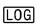or the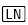key on your calculator. Solve each of the following problems, first using base 10 logs and then using natural logs. Homework Help ✎

1. 10,000(1.08)x = 20,000

2. 30,000(0.8)x = 15,000

3. Interpret the answer for part (a) if the equation represents an amount of money invested at 8% annual interest.

4. Interpret the answer to part (b) if the equation represents the price paid for a car that depreciates at 20% per year.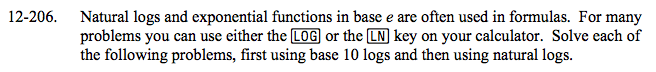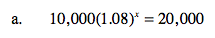log(1.08x) = log2

x · log(1.08) = log2

9.00646832

You should get the same answer using natural logs.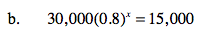See part (a).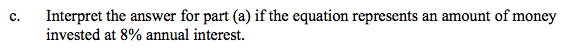What does x represent in this equation?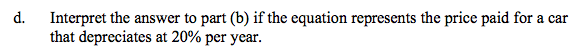What does x represent in this equation?